# 二、OpenCV 基础知识简介

• 使用 CMake 配置项目
• 从磁盘读取图像/向磁盘写入图像
• 阅读视频和访问摄像设备
• 主要图像结构(例如，矩阵)
• 其他重要和基本的结构(例如，矢量和标量)
• 基本矩阵运算入门
• 使用 XML/YAML 持久性 OpenCV API 进行文件存储操作

# 基本 CMake 配置文件

CMake 使用名为CMakeLists.txt的配置文件，其中定义了编译和依赖过程。 对于基于从单个源代码文件构建的可执行文件的基本项目，只需包含三行代码的CMakeLists.txt文件即可。 该文件如下所示：

cmake_minimum_required (VERSION 3.0)
project (CMakeTest)
add_executable(${PROJECT_NAME} main.cpp)  第一行定义了所需的 CMake 的最低版本。 此行在我们的CMakeLists.txt文件中是必需的，并允许我们使用特定版本中定义的 CMake 的功能；在我们的示例中，我们至少需要 CMake 3.0。 第二行定义项目名称。 此名称保存在名为PROJECT_NAME的变量中。 最后一行从main.cpp文件创建一个可执行命令(add_executable())，并为其提供与我们的项目(${PROJECT_NAME})相同的名称，并将源代码编译成名为CMakeTest的可执行文件，这是我们设置为项目名称的名称。 ${}表达式允许访问我们的环境中定义的任何变量。 然后，我们可以使用${PROJECT_NAME}变量作为可执行的输出名称。

# 创建库

CMake 允许我们创建 OpenCV 构建系统使用的库。 分解多个应用之间的共享代码是软件开发中常见且有用的做法。 在大型应用中，或者在多个应用中共享的公共代码中，这种做法非常有用。 在本例中，我们不创建二进制可执行文件，而是创建一个包含所有函数、类等的编译文件。 然后，我们可以与其他应用共享此库文件，而无需共享源代码。

# Create our hello library

# Create our application that uses our new library

# Link our executable with the new library


#开头的行添加注释，并被 CMake 忽略。 add_library*(Hello hello.cpp hello.h)命令定义库的源文件及其名称，其中Hello是库名，hello.cpphello.h是源文件。 我们还添加了头文件，以允许诸如 Visual Studio 之类的 IDE 链接到头文件。 此行将生成共享(.so用于 Mac OS X，Unix 或.dll用于 Windows)或静态库(.a用于 Mac OS X，Unix 或.lib用于 Windows)文件，具体取决于我们在库名和源文件之间添加的是SHARED还是STATIC字。 target_link_libraries(executable Hello)是将我们的可执行文件链接到所需库的函数，在我们的例子中，它是Hello库。

# 管理依赖项

CMake 能够搜索我们的依赖项和外部库，使我们能够根据项目中的外部组件构建复杂的项目，并添加一些需求。

    cmake_minimum_required (VERSION 3.0)
PROJECT(Chapter2)
# Requires OpenCV
FIND_PACKAGE( OpenCV 4.0.0 REQUIRED )
# Show a message with the opencv version detected
MESSAGE("OpenCV version : ${OpenCV_VERSION}") # Add the paths to the include directories/to the header files include_directories(${OpenCV_INCLUDE_DIRS})
# Add the paths to the compiled libraries/objects
link_directories(${OpenCV_LIB_DIR}) # Create a variable called SRC SET(SRC main.cpp) # Create our executable ADD_EXECUTABLE(${PROJECT_NAME} ${SRC}) # Link our library TARGET_LINK_LIBRARIES(${PROJECT_NAME} ${OpenCV_LIBS})  现在，让我们从以下几个方面来了解脚本的工作原理： cmake_minimum_required (VERSION 3.0) cmake_policy(SET CMP0012 NEW) PROJECT(Chapter2)  第一行定义了 CMake 的最低版本，第二行告诉 CMake 使用 CMake 的新行为来帮助识别正确的数字和布尔常量，而无需取消引用具有此类名称的变量；该策略是在 CMake 2.8.0 中引入的，当该策略未从 3.0.2 版开始设置时，CMake 会发出警告。 最后，最后一行定义了项目标题。 定义项目名称后，我们必须定义需求、库和依赖项： # Requires OpenCV FIND_PACKAGE( OpenCV 4.0.0 REQUIRED ) # Show a message with the opencv version detected MESSAGE("OpenCV version :${OpenCV_VERSION}")
include_directories(${OpenCV_INCLUDE_DIRS}) link_directories(${OpenCV_LIB_DIR})


The FIND_PACKAGE command includes all OpenCV submodules, but you can specify the submodules that you want to include in the project by executing your application smaller and faster. For example, if we are only going to work with the basic OpenCV types and core functionality, we can use the following command: FIND_PACKAGE(OpenCV 4.0.0 REQUIRED core).

OpenCV version : 4.0.0


${OpenCV_VERSION}是 CMake 存储 OpenCV 包版本的变量。include_directories()link_directories()将指定库的头和目录添加到我们的环境中。 OpenCV CMake 的模块将此数据保存在${OpenCV_INCLUDE_DIRS}${OpenCV_LIB_DIR}变量中。 并非所有平台(如 Linux)都需要这些行，因为这些路径通常位于环境中，但建议使用多个 OpenCV 版本来选择正确的链接并包含目录。 现在是将我们开发的资源包括在内的时候了： # Create a variable called SRC SET(SRC main.cpp) # Create our executable ADD_EXECUTABLE(${PROJECT_NAME} ${SRC}) # Link our library TARGET_LINK_LIBRARIES(${PROJECT_NAME} ${OpenCV_LIBS})  最后一行创建可执行文件，并将可执行文件与 OpenCV 库链接，正如我们在上一节创建库中所看到的那样。*这段代码中有一个新函数SET；该函数创建一个新变量，并向其添加我们需要的任何值。 在我们的示例中，我们在SRC变量中合并了main.cpp参数的值。 我们可以向同一变量添加越来越多的值，如以下脚本所示： SET(SRC main.cpp utils.cpp color.cpp )  # 使脚本更加复杂 在本节中，我们将展示一个更复杂的脚本，它包含子文件夹、库和可执行文件；总共只有两个文件和几行代码，如此脚本所示。 创建多个CMakeLists.txt文件不是强制性的，因为我们可以在主CMakeLists.txt文件中指定所有内容。 但是，对每个项目子文件夹使用不同的CMakeLists.txt文件更为常见，从而使其更加灵活和可移植。 此示例有一个代码结构文件夹，其中包含一个用于utils库的文件夹和一个根文件夹，根文件夹包含主可执行文件： CMakeLists.txt main.cpp utils/ CMakeLists.txt computeTime.cpp computeTime.h logger.cpp logger.h plotting.cpp plotting.h  然后，我们必须定义两个CMakeLists.txt文件，一个在根文件夹中，另一个在根文件夹中。 CMakeLists.txt根文件夹文件包含以下内容：  cmake_minimum_required (VERSION 3.0) project (Chapter2) # Opencv Package required FIND_PACKAGE( OpenCV 4.0.0 REQUIRED ) #Add opencv header files to project include_directories(${OpenCV_INCLUDE_DIR})
link_directories(${OpenCV_LIB_DIR}) # Add a subdirectory to the build. add_subdirectory(utils) # Add optional log with a precompiler definition option(WITH_LOG "Build with output logs and images in tmp" OFF) if(WITH_LOG) add_definitions(-DLOG) endif(WITH_LOG) # generate our new executable add_executable(${PROJECT_NAME} main.cpp)
# link the project with his dependencies
target_link_libraries(${PROJECT_NAME}${OpenCV_LIBS} Utils)


utils个文件夹的CMakeLists.txt个文件中，我们将编写一个新的库以包括在我们的主项目文件夹中：

# Add new variable for src utils lib
SET(UTILS_LIB_SRC
computeTime.cpp
logger.cpp
plotting.cpp
)
# create our new utils lib
add_library(Utils ${UTILS_LIB_SRC}) # make sure the compiler can find include files for our library target_include_directories(Utils PUBLIC${CMAKE_CURRENT_SOURCE_DIR})


#ifdef LOG
logi("Number of iteration %d", i);
#endif


if(WITH_LOG)
endif(WITH_LOG)


# 图像和矩阵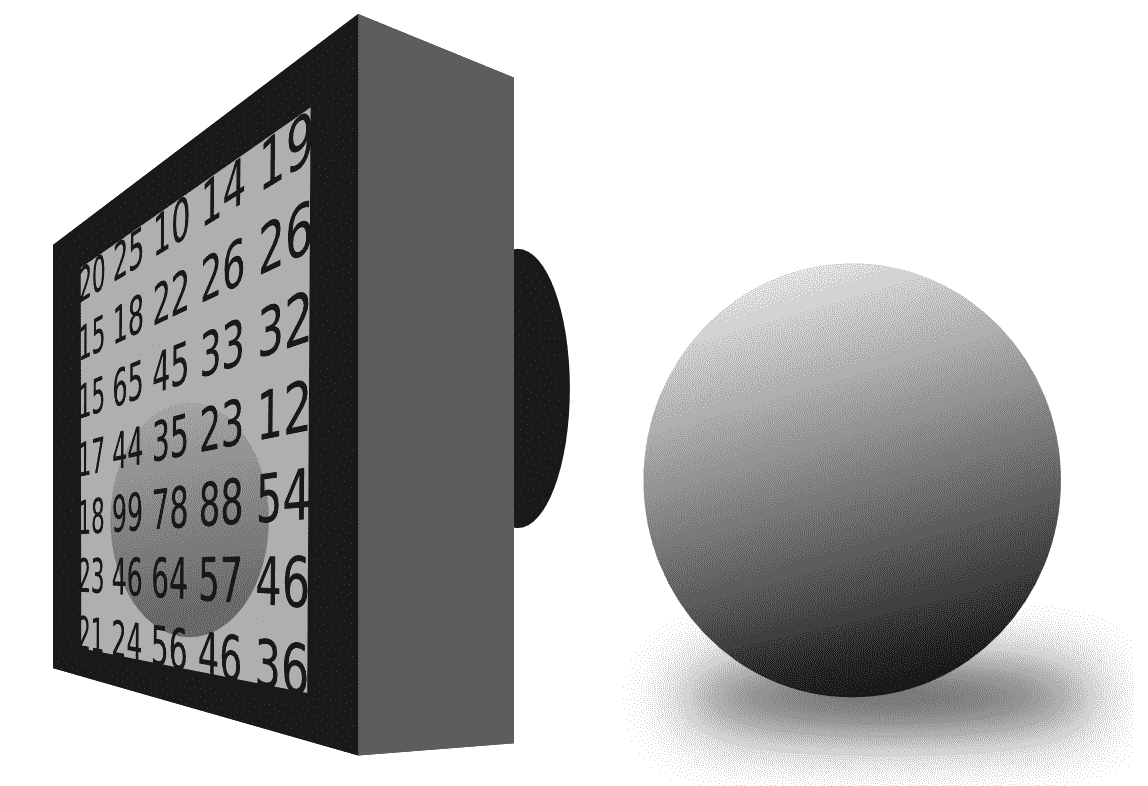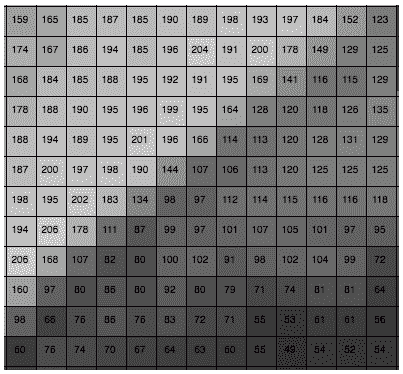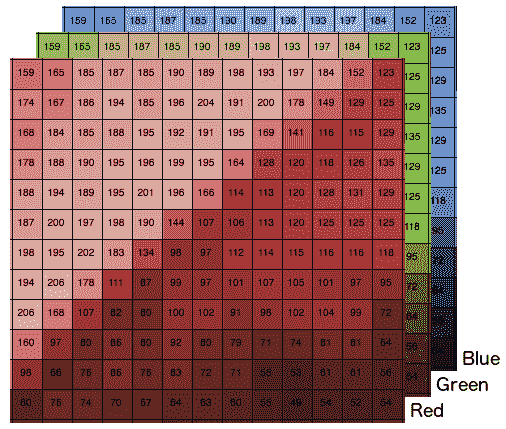| 第 0 行 | 第 1 行 | 第 2 行 | | 使用 0 | 使用 1 个 | 使用 2 个 | 使用 0 | 使用 1 个 | 使用 2 个 | 使用 0 | 使用 1 个 | 使用 2 个 | | 像素ρ1 | 像素 2 | 像素 3 | 像素 4 | 像素 5 | 像素 6 | 像素 7 | 像素 8 | 像素 9 | | 英语字母表中第二个字母 / B 音 / 乙等 | 英语字母表中第七个字母 / 第七列 | 英语字母中的第十八个字母 / R 类 | 英语字母表中第二个字母 / B 音 / 乙等 | 英语字母表中第七个字母 / 第七列 | 英语字母中的第十八个字母 / R 类 | 英语字母表中第二个字母 / B 音 / 乙等 | 英语字母表中第七个字母 / 第七列 | 英语字母中的第十八个字母 / R 类 | 英语字母表中第二个字母 / B 音 / 乙等 | 英语字母表中第七个字母 / 第七列 | 英语字母中的第十八个字母 / R 类 | 英语字母表中第二个字母 / B 音 / 乙等 | 英语字母表中第七个字母 / 第七列 | 英语字母中的第十八个字母 / R 类 | 英语字母表中第二个字母 / B 音 / 乙等 | 英语字母表中第七个字母 / 第七列 | 英语字母中的第十八个字母 / R 类 | 英语字母表中第二个字母 / B 音 / 乙等 | 英语字母表中第七个字母 / 第七列 | 英语字母中的第十八个字母 / R 类 | 英语字母表中第二个字母 / B 音 / 乙等 | 英语字母表中第七个字母 / 第七列 | 英语字母中的第十八个字母 / R 类 | 英语字母表中第二个字母 / B 音 / 乙等 | 英语字母表中第七个字母 / 第七列 | 英语字母中的第十八个字母 / R 类 |

Value= Row_i*num_cols*num_channels + Col_i + channel_i


OpenCV functions are quite optimized for random access, but sometimes, direct access to the memory (work with pointer arithmetic) is more efficient, for example, when we have to access all pixels in a loop.

# 读/写图像

#include <iostream>
#include <string>
#include <sstream>
using namespace std;

// OpenCV includes
#include "opencv2/core.hpp"
#include "opencv2/highgui.hpp"
using namespace cv;

int main(int argc, const char** argv)
{

if(! color.data ) // Check for invalid input
{
cout << "Could not open or find the image" << std::endl ;
return -1;
}
// Write images
imwrite("lenaGray.jpg", gray);

// Get same pixel with opencv function
int myRow=color.cols-1;
int myCol=color.rows-1;
Vec3b pixel= color.at<Vec3b>(myRow, myCol);
cout << "Pixel value (B,G,R): (" << (int)pixel << "," << (int)pixel << "," << (int)pixel << ")" << endl;

// show images
imshow("Lena BGR", color);
imshow("Lena Gray", gray);
// wait for any key press
waitKey(0);
return 0;
}



// OpenCV includes
#include "opencv2/core.hpp"
#include "opencv2/highgui.hpp"
using namespace cv;


// Read images


imread是读取图像的主要功能。 此函数用于打开图像并以矩阵格式存储。 imread接受两个参数。 第一个参数是包含图像路径的字符串，第二个参数是可选的，默认情况下将图像加载为彩色图像。 第二个参数允许以下选项：

• cv::IMREAD_UNCHANGED：如果设置，则在输入具有相应深度时返回 16 位/32 位图像，否则将其转换为 8 位
• cv::IMREAD_COLOR：如果设置，则始终将图像转换为彩色图像(BGR，8 位无符号)
• cv::IMREAD_GRAYSCALE：如果设置，则始终将图像转换为灰度图像(8 位无符号)

// Write images
imwrite("lenaGray.jpg", gray);


// Get same pixel with opencv function
int myRow=color.cols-1;
int myCol=color.rows-1;


Vec3b pixel= color.at<Vec3b>(myRow, myCol);
cout << "Pixel value (B,G,R): (" << (int)pixel << "," << (int)pixel << "," << (int)pixel << ")" << endl;


// show images
imshow("Lena BGR", color);
imshow("Lena Gray", gray);
// wait for any key press
waitKey(0);


If we want to stop the application from waiting, we can use the OpenCV function waitKey, with a parameter of the number of milliseconds we want to wait for a key press. If we set up the parameter to 0, then the function will wait until a key is pressed.cmake_minimum_required (VERSION 3.0)
cmake_policy(SET CMP0012 NEW)
PROJECT(project)

# Requires OpenCV
FIND_PACKAGE( OpenCV 4.0.0 REQUIRED )
MESSAGE("OpenCV version : ${OpenCV_VERSION}") include_directories(${OpenCV_INCLUDE_DIRS})
link_directories(${OpenCV_LIB_DIR}) ADD_EXECUTABLE(sample main.cpp) TARGET_LINK_LIBRARIES(sample${OpenCV_LIBS})


1. 创建一个build文件夹。
2. build文件夹内，执行 CMake 或在 Windows 中打开 CMake GUI 应用，选择sourcebuild文件夹，然后按配置和生成按钮。
3. 如果您使用的是 Linux 或 MacOS，请像往常一样生成一个 Makefile，然后使用*make命令编译该项目。 如果您使用的是 Windows，请使用在步骤 2 中选择的编辑器打开项目，然后编译。

# 阅读视频和摄像机

// OpenCV command line parser functions
// Keys accepted by command line parser
const char* keys =
{
"{help h usage ? | | print this message}"
"{@video | | Video file, if not defined try to use webcamera}"
};


"{name_param | default_value | description}"


name_param前面可以有@，它将此参数定义为默认输入。 我们可以使用多个name_param

CommandLineParser parser(argc, argv, keys);


//If requires help show
if (parser.has("help"))
{
parser.printMessage();
return 0;
}


.has类方法检查参数是否存在。 在示例中，我们检查用户是否添加了参数help?，然后使用类函数printMessage显示所有描述参数：

   String videoFile= parser.get<String>(0);


   // Check if params are correctly parsed in his variables
if (!parser.check())
{
parser.printErrors();
return 0;
}


VideoCapture cap; // open the default camera
if(videoFile != "")
cap.open(videoFile);
else
cap.open(0);
if(!cap.isOpened())  // check if we succeeded
return -1;


namedWindow("Video",1);
for(;;)
{
Mat frame;
cap >> frame; // get a new frame from camera
if(frame)
imshow("Video", frame);
if(waitKey(30) >= 0) break;
}
// Release the camera or video cap
cap.release();


The time required to wait for the next frame using camera access is calculated from the camera speed and our spent algorithm time. For example, if a camera works at 20 fps, and our algorithm spent 10 milliseconds, a great waiting value is 30 = (1000/20) - 10 milliseconds. This value is calculated considering a wait of a sufficient amount of time to ensure that the next frame is in the buffer. If our camera takes 40 milliseconds to take each image, and we use 10 milliseconds in our algorithm, then we only need to stop with waitKey 30 milliseconds, because 30 milliseconds of wait time, plus 10 milliseconds of our algorithm, is the same amount of time for which each frame of the camera is accessible.

It is very important to release all resources that we use in a computer vision application. If we do not, we can consume all RAM memory. We can release the matrices using the release function.

# 其他基本对象类型

• Vec
• Scalar
• Point
• Size
• Rect
• RotatedRect

# VEC 对象类型

Vec是一个主要用于数值向量的模板类。 我们可以定义任何类型的向量和分量数量：

Vec<double,19> myVector;


typedef Vec<uchar, 2> Vec2b;
typedef Vec<uchar, 3> Vec3b;
typedef Vec<uchar, 4> Vec4b;

typedef Vec<short, 2> Vec2s;
typedef Vec<short, 3> Vec3s;
typedef Vec<short, 4> Vec4s;

typedef Vec<int, 2> Vec2i;
typedef Vec<int, 3> Vec3i;
typedef Vec<int, 4> Vec4i;

typedef Vec<float, 2> Vec2f;
typedef Vec<float, 3> Vec3f;
typedef Vec<float, 4> Vec4f;
typedef Vec<float, 6> Vec6f;

typedef Vec<double, 2> Vec2d;
typedef Vec<double, 3> Vec3d;
typedef Vec<double, 4> Vec4d;
typedef Vec<double, 6> Vec6d;



All the following vector operations are also implemented: v1 = v2 + v3 v1 = v2 - v3 v1 = v2 * scale v1 = scale * v2 v1 = -v2 v1 += v2

# 标量对象类型

Scalar对象类型是从Vec派生的具有四个元素的模板类。 Scalar类型在 OpenCV 中广泛用于传递和读取像素值。

Scalar s0(0);
Scalar s1(0.0, 1.0, 2.0, 3.0);
Scalar s2(s1);


# 点对象类型

Like Point, there is a Point3 template class for 3D point support.

Vec类一样，为方便起见，OpenCV 定义了以下Point别名：

typedef Point_<int> Point2i;
typedef Point2i Point;
typedef Point_<float> Point2f;
typedef Point_<double> Point2d;
The following operators are defined for points:
pt1 = pt2 + pt3;
pt1 = pt2 - pt3;
pt1 = pt2 * a;
pt1 = a * pt2;
pt1 = pt2 / a;
pt1 += pt2;
pt1 -= pt2;
pt1 *= a;
pt1 /= a;
double value = norm(pt); // L2 norm
pt1 == pt2;
pt1 != pt2;


# 大小对象类型

OpenCV 中另一个非常重要且广泛使用的模板类是用于指定图像或矩形大小的模板类Size。 该类添加了两个成员：width 和 Height，以及有用的函数 Sizearea()。*在下面的示例中，我们可以看到使用 SIZE 的多种方法：

Size s(100,100);
Mat img=Mat::zeros(s, CV_8UC1); // 100 by 100 single channel matrix
s.width= 200;
int area= s.area(); returns 100x200


# 矩形对象类型

Rect是定义由以下参数定义的 2D 矩形的另一个重要模板类：

• 左上角的坐标

• 矩形的宽度和高度

Rect模板类可用于定义图像的感兴趣区域和(ROI)，如下所示：

Mat img=imread("lena.jpg");
Rect rect_roi(0,0,100,100);
Mat img_roi=img(r);


# RotatedRect 对象类型

RotatedRect(const Point2f& center, const Size2f& size, float angle);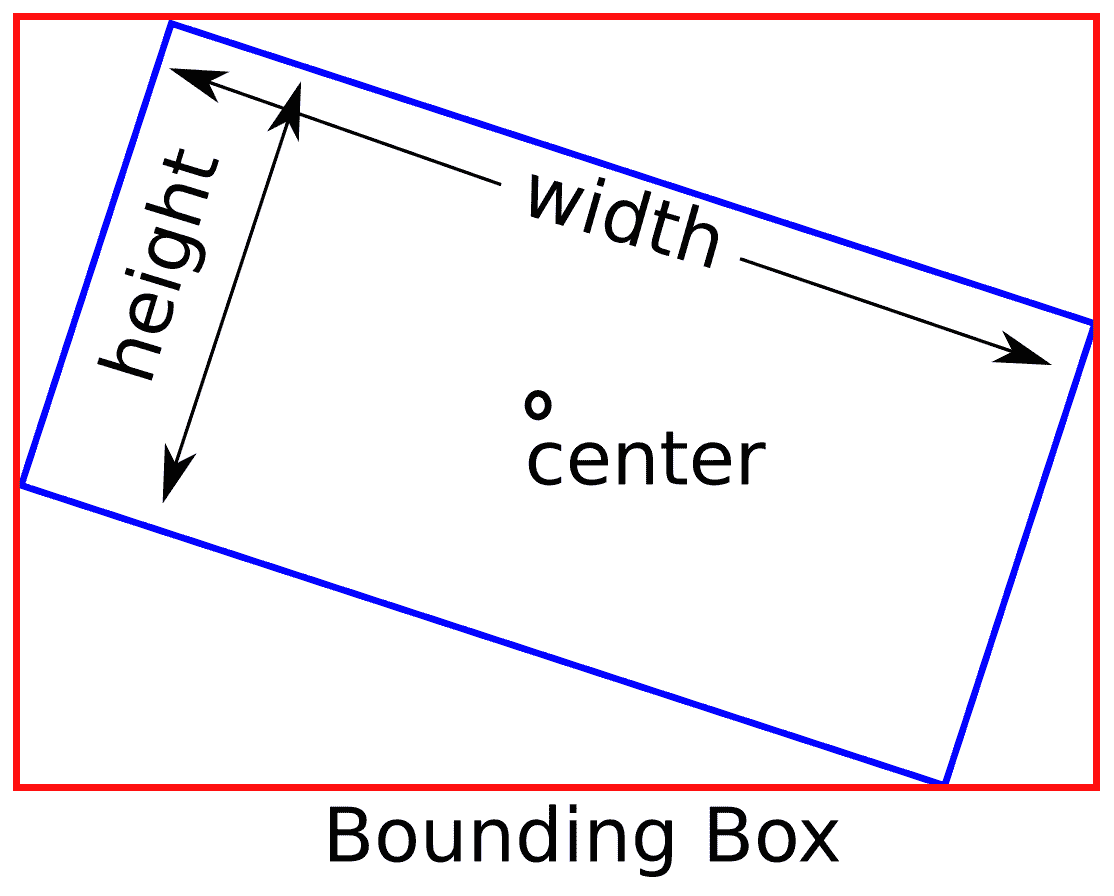# 基本矩阵运算

Mat a= Mat(Size(5,5), CV_32F);


You can create a new matrix linking with a stored buffer from third-party libraries without copying data using this constructor: Mat(size, type, pointer_to_buffer).

CV_8UC1
CV_8UC3
CV_8UC4
CV_32FC1
CV_32FC3
CV_32FC4


You can create any type of matrix using CV_number_typeC(n), where the number_type is 8 bits unsigned (8U) to 64 float (64F), and where (n) is the number of channels; the number of channels permitted ranges from 1 to CV_CN_MAX.

Mat mz= Mat::zeros(5,5, CV_32F);
Mat mo= Mat::ones(5,5, CV_32F);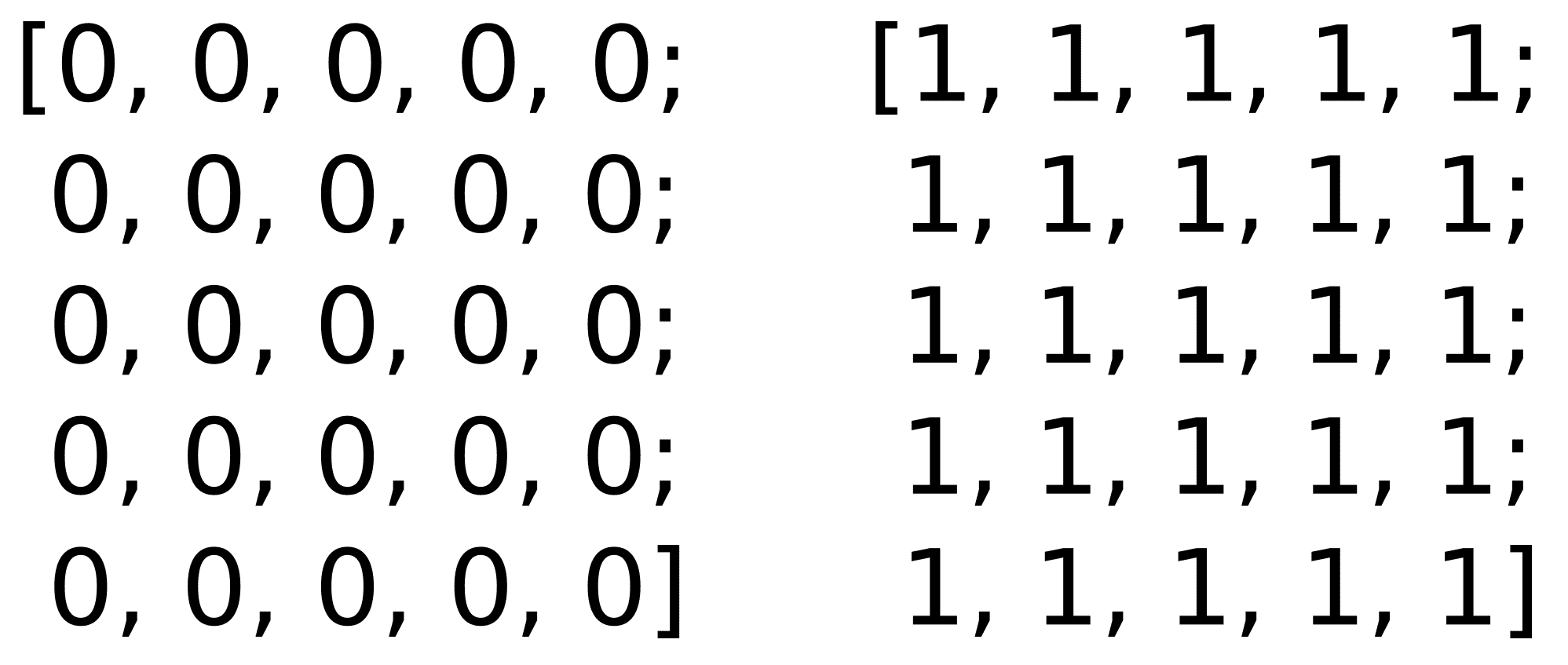Mat m= Mat::eye(5,5, CV_32F);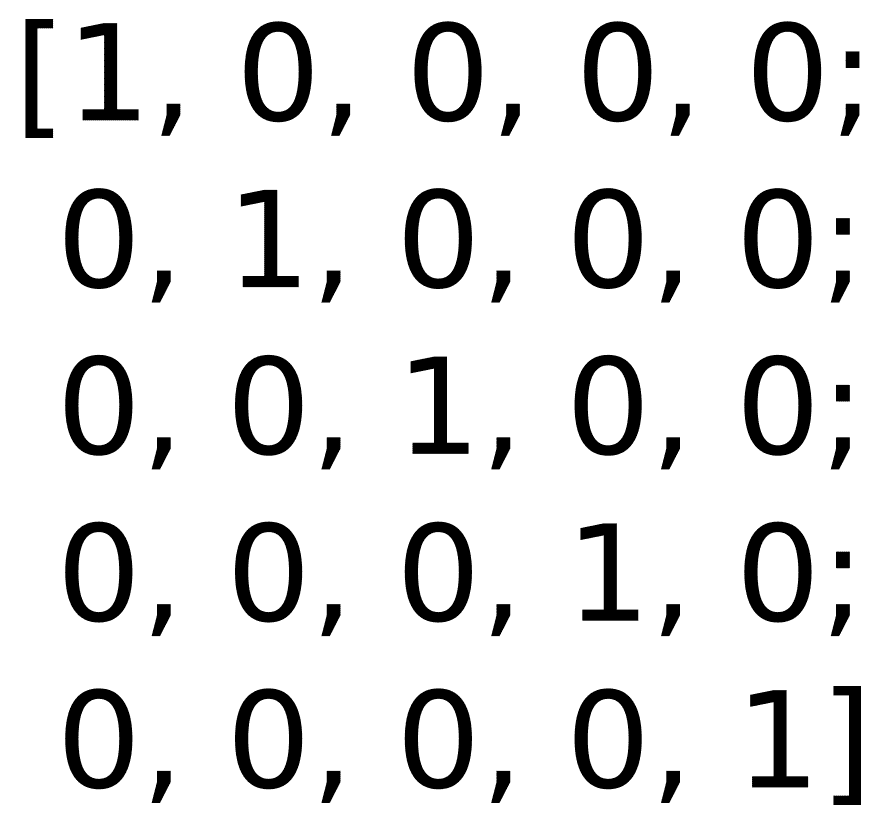OpenCV 的Mat类中允许所有矩阵运算。 我们可以使用+-运算符将两个大小相同的矩阵相加或相减，如以下代码块所示：

Mat a= Mat::eye(Size(3,2), CV_32F);
Mat b= Mat::ones(Size(3,2), CV_32F);
Mat c= a+b;
Mat d= a-b;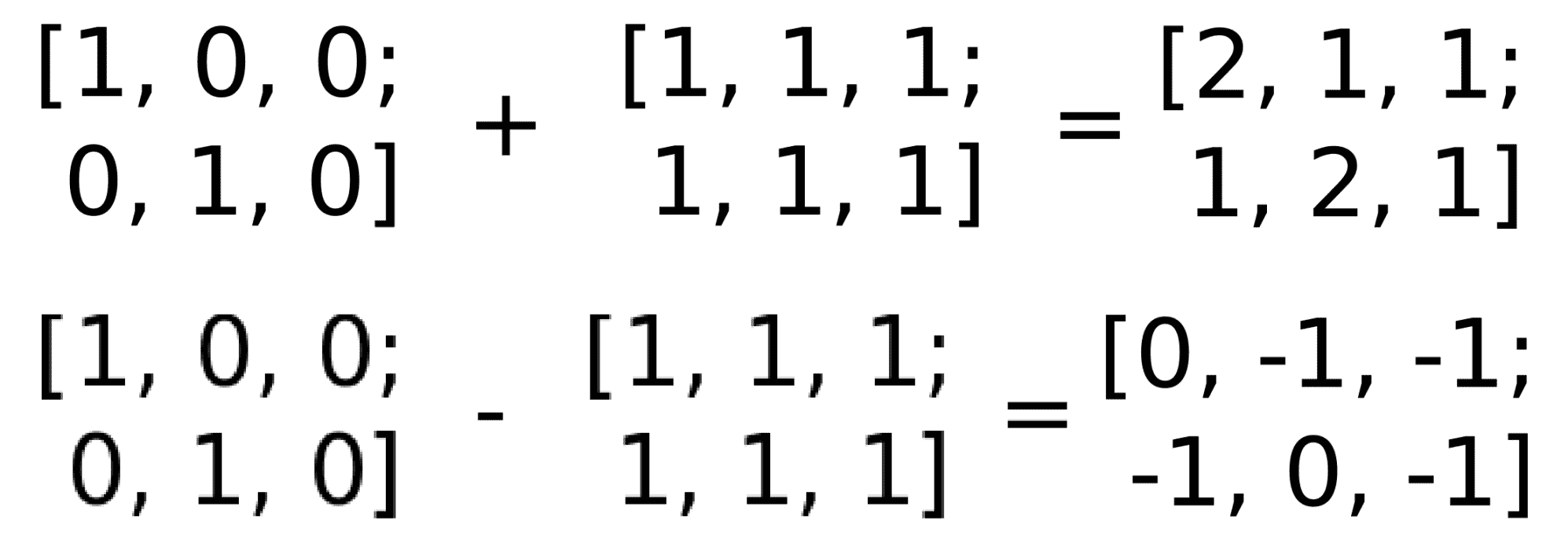Mat m1= Mat::eye(2,3, CV_32F);
Mat m2= Mat::ones(3,2, CV_32F);
// Scalar by matrix
cout << "nm1.*2n" << m1*2 << endl;
// matrix per element multiplication
cout << "n(m1+2).*(m1+3)n" << (m1+1).mul(m1+3) << endl;
// Matrix multiplication
cout << "nm1*m2n" << m1*m2 << endl;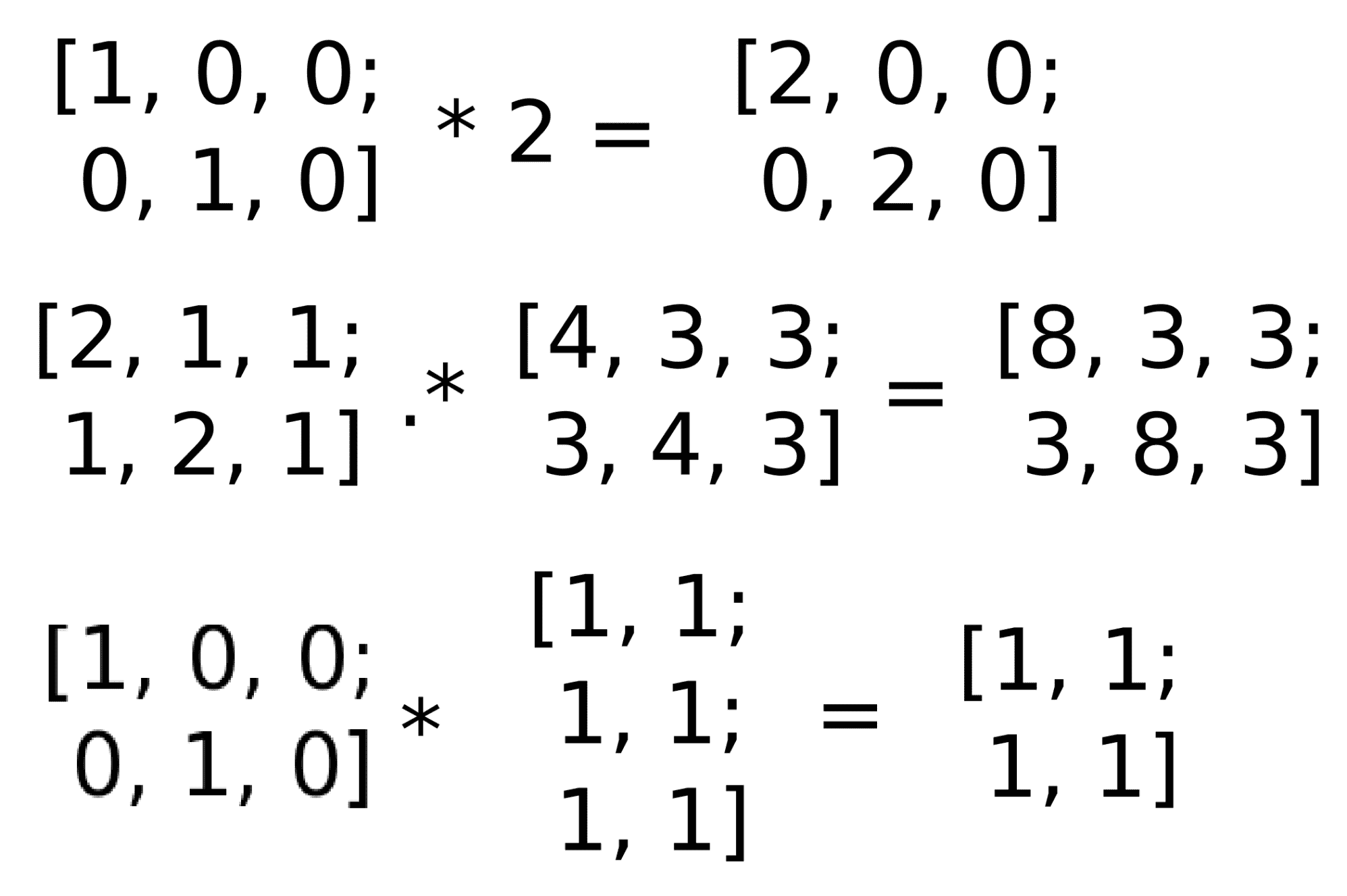int countNonZero(src);


OpenCV 提供了一些统计功能。 通道的平均值和标准偏差可以使用以下函数meanStdDev计算：

meanStdDev(src, mean, stddev);


minMaxLoc(src, minVal, maxVal, minLoc, maxLoc);


Other core and useful functions are described in detail at: http://docs.opencv.org/modules/core/doc/core.html.

# 写入文件存储

#include "opencv2/opencv.hpp"
using namespace cv;

int main(int, char** argv)
{
// create our writer
FileStorage fs("test.yml", FileStorage::WRITE);
// Save an int
int fps= 5;
fs << "fps" << fps;
// Create some mat sample
Mat m1= Mat::eye(2,3, CV_32F);
Mat m2= Mat::ones(3,2, CV_32F);
Mat result= (m1+1).mul(m1+3);
// write the result
fs << "Result" << result;
// release the file
fs.release();

Mat r;
fs2["Result"] >> r;
std::cout << r << std::endl;

fs2.release();

return 0;
}


FileStorage fs("test.yml", FileStorage::WRITE);


int fps= 5;
fs << "fps" << fps;


Mat m1= Mat::eye(2,3, CV_32F);
Mat m2= Mat::ones(3,2, CV_32F);
Mat result= (m1+1).mul(m1+3);
// write the result
fs << "Result" << result;


%YAML:1.0
fps: 5
Result: !!opencv-matrix
rows: 2
cols: 3
dt: f
data: [ 8., 3., 3., 3., 8., 3\. ]


#include "opencv2/opencv.hpp"
using namespace cv;

int main(int, char** argv)
{

Mat r;
fs2["Result"] >> r;
std::cout << r << std::endl;

fs2.release();

return 0;
}


FileStorage fs2("test.yml", FileStorage::READ);


Mat r;
fs2["Result"] >> r;